# Chapter 1f: Redox reactions

Oxido-reductions, or redox, are reactions involving a transfer of charge between molecules.  During such reactions, some chemical energy is transformed into electrical energy.

An oxidation reaction is a reaction during which a substrate (molecule, atom or ion) loses electrons.

A reduction reaction is a reaction during which a substrate gains electrons.

An oxidant is a substrate with the ability to oxidize other substances. During this process, the oxidant is thus reduced. They are also called oxidizer or oxidizing agent.

A reductant is a substrate with the ability to reduce other substances. During this process, the reductant is thus oxidized. They are also called reducer or reducing agent.

The state of oxidation

The state of oxidation (SO) is an integer the value of which is the charge of an atom if we break all its liaisons. In O2, each atom takes its electrons back (it is a homolytic cleavage or homolysis). The global charge of O2 is neutral. Thus the SO of each oxygen is 0. However, in H2O, the oxygen takes the electrons from the hydrogen’s when we break the liaisons because the oxygen is more electronegative than the hydrogen (it is a heterolytic cleavage or heterolysis). The SO of oxygen in H2O is -2 and the SO of the hydrogen’s is +1.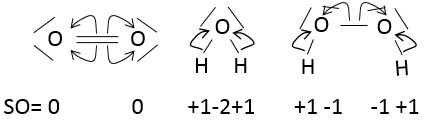In general, the SO of the oxygen is -2 and we can find the SO of the other atoms of the molecule without the full representation of the molecule. For example, we can determine the state of oxidation of the manganese in MnO4: the global charge is -1 and each oxygen has a SO of -2. The SO of Mn is thus +7 so that the global charge equals the sum of the states of oxidation -1=+7-(4x-2). In heavy water, H2O2, the SO of the oxygen’s is -1.

An atom can thus have several possible SO. If several SO are present for one atom in a single molecule, we take the average between them. For example, S has a SO of +2.5 in S4O62-. Sulphur can have a SO from 0 (solid sulphur) to +6 (H2SO4).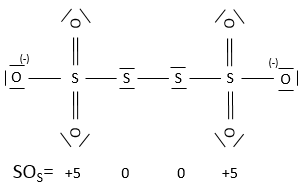Redox reactions

So, in a redox reaction, an oxidant oxidizes a reductant while the reductant reduces the oxidant. In presence of two compounds, it is however not always obvious to determine the direction of the reaction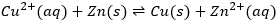This reaction involves two half reactions: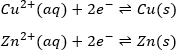The reaction will go in the direction that has a negative enthalpy. In the present case, the reaction goes from left to right and it can be explained because Cu is more electronegative than Zn. It is thus harder to take its electron from Cu than from Zn.

It is possible to determine the strength of the oxidants and reductants from their ability to attract electrons in a battery, i.e. their standard potential ε0 (in volts). However, an absolute value of the standard potential is not measured. We can only know the value of ε0 with regard to another couple. We use the couple H+/H2 as a reference, with its standard potential set to ε0=0.000V in sctp by convention. The name of a couple is put in the direction of the reduction: oxidant/reductant. For example: Cu2+/Cu, Zn2+/Zn.

To establish a redox reaction correctly, a method exists:

First, we write the supposed equation without stoechiometry, protons, water or OH. Just the oxidants and reductants. We determine their state of oxidation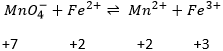Second, we determine the individual standard potentials of each couple. The values of ε0 can be found for a large range of compounds on the back of Mendeleev tables. It is given for the reduction reactions (for the oxidation, take the negative value). For the iron, the reaction is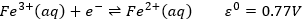For the manganese, 5 electrons are added to obtain Mn2+.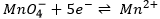Be careful to consider how many atoms are reduced/oxidized. In the couple Cr2O72-/Cr3+, Cr has a SO of +6→+3 but 6 electrons have to be added in the reaction because two Cr are reduced.

Coming back to the problem involving manganese, the reaction is not balanced yet. Oxygen’s are also involved in the process. We balance the reaction with water molecules, protons or OH depending on the acidity of the solution. To determine how many species are required, we count the charges at each side of the arrow: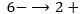There are 6- at the left because MnO4- is negatively charged. The difference of 8 charges is balanced by the addition of 8 protons at the left of the arrow and we balance the equation: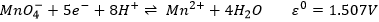The standard potential of this reaction is known. Now, we put together the two half reactions. Don’t forget to consider that one half reaction introduces 5 electrons and the other one introduces 1 electrons. The equation of Fe3+/Fe2+ is thus multiplied by 5.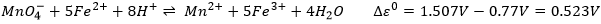This final reaction is correctly balanced. Note that even if the half reaction Fe3+/Fe2+ is multiplied by 5, it is not multiplied in the determination of the standard potential. As Δε0>0, it means that the reactions goes from left to right. This reaction is spontaneous if the production of enthalpy ΔG0<0. The enthalpy of reaction is a measure of the work required to do the reaction. If this value is negative, the reaction is spontaneous and liberates energy, generally in the form of heat. The reaction is said to be exothermic. If this value is positive, it is necessary to give some energy to do the reaction. The reaction is endothermic as it absorbs heat from its surrounding.

The relation between the enthalpy of a reaction and its potential is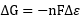This relations comes from the fact that a potential V is the variation of work J to modify the charge Q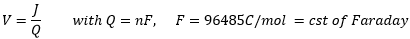Batteries

In batteries, the elements of a redox reactions are separated. The two solutions are connected by a salt bridge and two electrodes connected to a voltmeter.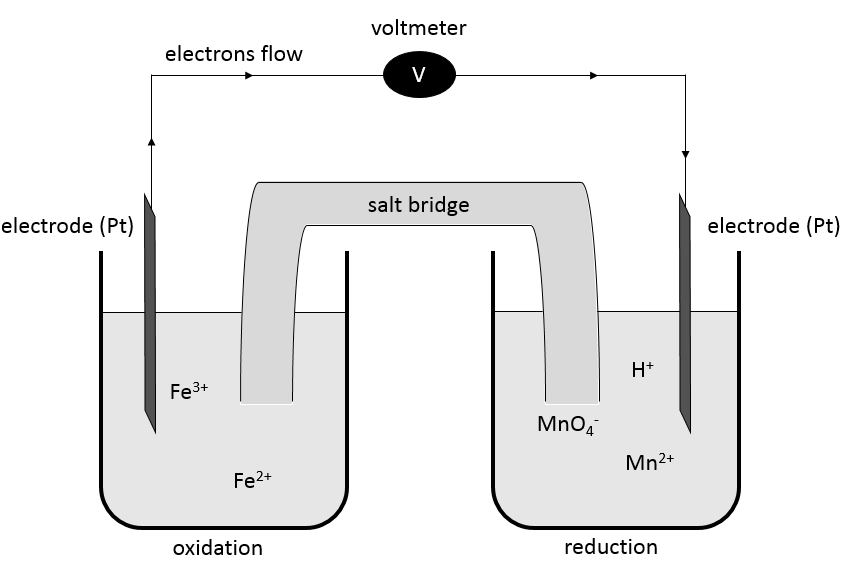A salt bridge is a device used to connect two half batteries, that is full of ionic species conducting electricity (electrolytes) but not interfering with the compounds of the battery. Without the salt bridge, one half cell would accumulate negative charge and the other half cell would accumulate positive charge as the reaction proceeds. The cations and anions of the half bridge are chosen so that they have a similar high conductivity. KCl, KNO3, NH4Cl are some examples of electrolytes composing a salt bridge.

A battery is written by convention as follows: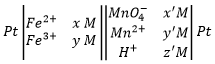The electrodes are put at the extremities and the compounds of the two half batteries are separated by a double line symbolizing the salt bridge. The oxidation is put in first and the reduction in second.

The oxidation of Fe2+ is done at the electrode of platinum that catches the freed electron. This electron goes through the voltmeter to the other half battery where it is used in the reduction. The salt bridge closes the electric circuit.

The potential of a battery, also called electromotive force, is given by the Nermst equation and depends on the concentrations of the different species: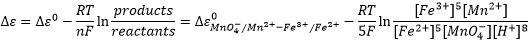We can also obtain the potential from the difference between the two cells of the battery: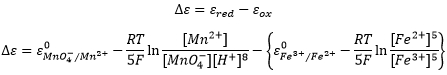Be careful that the concentrations of Fe2+ and Fe3+ were inverted in the last equation because we consider the products and reactants of the half batteries in this case.

In a battery of concentration, the electromotive force is only given by the concentrations of the species: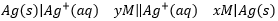The two sides of the battery have the same ε0 and the equation for the electromotive force is thus limited to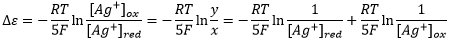Here, we cannot use KCl as electrolyte because Cl would react with Ag+ to form a precipitate of AgCl.

Electrodes of reference

An electrode of reference is an electrode the potential of which is known and does not varies during an experiment. The standard hydrogen electrode (SHE) is a first electrode of reference but is not often used because it is not entirely reproducible.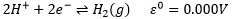Dihydrogen gas is introduced in an acid solution of 1M with a pressure of 1atm.

The silver chloride electrode is used as electrode of reference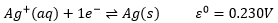The potential of the electrode depends on the concentration of the ionic form of Ag.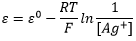However, this concentration also depends on the solubility of AgCl in water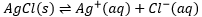The constant of dissolution of AgCl is KS=[Ag+][Cl]. By saturating the solution with KCl (>3.6M), the concentration of chloride is constant, hence the fixed concentration of Ag+.

The potential written above is not totally correct because at large concentrations, the concentration is no more equal to the activity of an ion.

The saturated calomel electrode uses the same principle: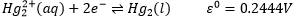Here the mercury cations precipitate with chloride which is saturated the same way as for the silver chloride electrode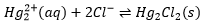The reverse of a battery is the electrolyse: a current is applied in a cell to induce a reaction with a negative electromotive force. It is a way to depose metals from a solution.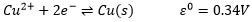The quantity of deposed metal depends on the applied current: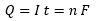All the first column of the Mendeleev table are obtained by electrolyse. In the nature, they exist in their oxidised form because the world is oxidant: H2O and O2 are everywhere. The production of Na can be done from its salt by electrolyse: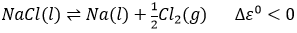This reaction is performed at high temperature (>600°C) and in total absence of water.

Disproportionation

In some cases, a single species plays simultaneously the role of oxidant and reductant. A reaction involving such a process is called disproportionation. The salt of copper dissociates in water in Cu+ and Cl-. However solid copper is obtained during the process. It is a consequence of the disproportionation of copper: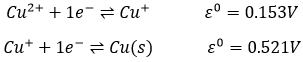As the second reaction has a larger potential, the global reactions forms solid copper: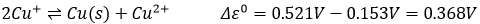The Cu+ dissociated from CuCl forms spontaneously Cu2+ and Cu(s).

Monoxide of carbon is the result of disproportionation between CO2 and C. It is a process responsible of many incidents, often lethal, in bathrooms with an insufficient ventilation. Traces of carbon, obtained from burned organic compounds, reacts with the carbon dioxide emitted by boilers or heaters. The problem is that CO takes the place of O2 on our blood cells and is much strongly bound to them than O2 (by a factor 200). Once CO is bound to a blood cell, O2 can difficulty bind, Because of that, only a small proportion of CO can be catastrophic. To treat people affected by CO, they are placed in a room overpressured with O2 to force the equilibrium and remove the carbon monoxide.

The standard potential of a reaction can be found from its intermediate reactions.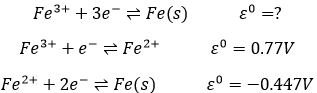In total, 3 electrons are required to obtain the iron on its solid state. 1 electron is required for the first step and 2 for the second step. The (approximate) standard potential is found by a combination of the standard potential of each step, taking in account the required electrons for each step: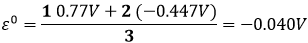In total, the process has a negative standard potential. The reverse reaction is thus spontaneous in presence of an oxidant. It is the production of stain (hydrated Fe3+) from iron. On a car for example, the iron is protected by a thin layer of painting/coating, not allowing water to the contact of the iron. If there is a default on the coating, the iron is oxidised but the stain will not always appears at this location: the electrons freed by the oxidation can move through the metal.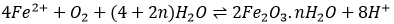To avoid this, surfaces are connected by an electric cable to a piece of zinc. The zinc will oxidise instead of the iron because its standard potential is smaller.

Stain is a porous material and the oxygen can pass through it to proceed further. ZnO is not porous so a monolayer of Zn can protect iron pieces from stain. It is the principle of stainless iron, but applied with Cr2O3.